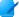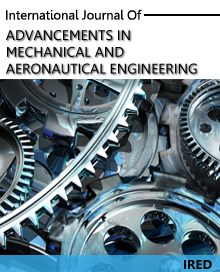# Journals Proceedings

International Journal of Advancements in Mechanical and Aeronautical Engineering

#### Analysis Of Cold Expanded Fastener Holes- FEM Approach

Author(s) : MAANASA BHAT    , PAURAV SARDESHMUKH    , SHANMUKHA NAGARAJ    , SRIRANJINI.K.SAbstract

Pre Stressing is a process of introducing residual compressive stresses zone around fastener holes which minimizes adverse effects of cyclic tensile stresses and retards the growth of fatigue cracks originating from the material flaws or surface imperfections. The present investigation is aimed at optimizing the inter hole distance between two adjacent cold expanded holes on a plate, limiting deformation of the material between holes to the elastic range by applying stress below the compressive yield strength of the material. It is found that ratio of central distance between holes to diameter of the holes (C/D) has a significant effect on the stress contours induced for a given percentage of expansion. The plate is modelled as a thick cylinder and equations are derived for radial stress. Graphs for these equations are plotted in MATLAB. ANSYS is used to conduct finite element analysis (FEA) of the cold expanded Mild Steel plate with two holes. To find the optimum centre distance between the two holes, the distance between the two holes was varied from 2D to 3.6D in steps of 0.2D and its effects, studied. The stress patterns in the vicinity of the holes from the analytical and Finite Element Methods were found to be comparable, proving the correctness of the approach. The relationship between C/D and percentage of expansion is analyzed, thus, optimizing the percentage of expansion required for a given C/D.

No fo Author(s) : 4
Page(s) : 71 - 75
Electronic ISSN : 2372-4153
Volume 1 : Issue 2